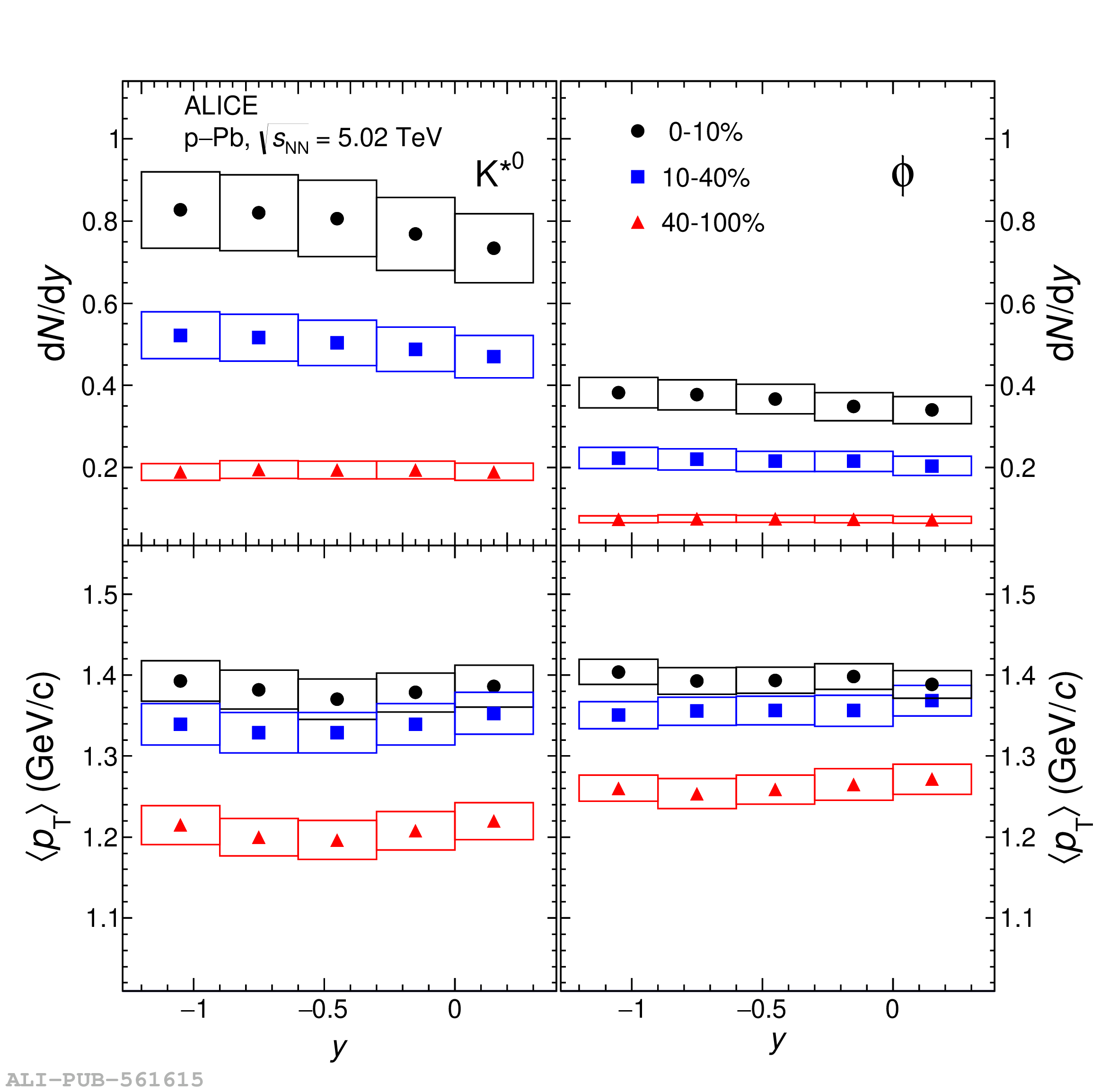# Figure A.1

 The \pt integrated yield (d$N/\mathrm{d}y$) (top panels) and mean transverse momentum ($\langle p_{\mathrm{T}} \rangle$) (bottom panels) for \kstar (left panels) and \phim (right panels) mesons as a function of $y$ measured for the multiplicity classes 0--10$\%$, 10--40$\%$ and 40--100$\%$ in \pPb collisions at \snn $=$ 5.02 TeV. The statistical uncertainties are represented as bars whereas boxes indicate the total systematic uncertainties on the measurements.